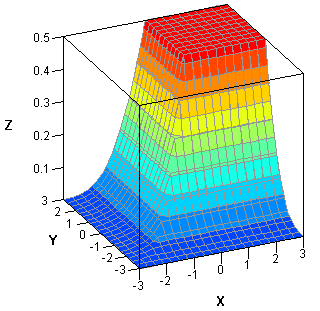Last week I received a message from SAS Technical Support saying that a customer's IML program was running slowly. Could I look at it to see whether it could be improved?

What I discovered is a good reminder about the importance of vectorizing user-defined modules. The program in this blog post is not the customer's, but it illustrates the main ideas that I'd like to discuss. Consider the following SAS/IML module:

```proc iml; start MinCDF(x, y); a = min(x, y); p = cdf("Normal", a); z = min(p, 0.5); return(z); finish;```

The function takes two scalar arguments, x and y, and does the following:

• It assigns the smaller of x and y to the variable a.
• It calls the CDF function to compute the probability that a normal random variable will be observed to have a value less than or equal to a. Numerically, this requires evaluating the area under the normal density curve from minus infinity to a.
• It returns that probability or 0.5, whichever is smaller.

The details of the function are not important. The important facts are

• It uses the MIN function to return the smaller of two scalar values.
• It performs a computationally expensive operation. In this case, the CDF call.
• It is a scalar function: each argument represents a scalar value and it returns a scalar value.The function is not vectorized, which means that you cannot pass in two vectors for x and y and get back a vector of results. (Try it!) The problem is the MIN function, which returns the smallest value (a scalar) from among all elements of x and y.

If you want to call the function on many pairs of x and y values, you have two options: call the function in a loop or rewrite it so that it will return a vector of results when passed vectors for input arguments. For example, you might want to evaluate the function on a fine grid of values in order to visualize the function as a surface over the xy-plane, as shown to the left. The following statements evaluate the function in a loop. The loop calls the function more than 360,000 times, and takes about a second:

```xy = ExpandGrid( do(-3,3,0.01), do(-3,3,0.01) ); /* grid of (x,y) values */ x = xy[,1]; y = xy[,2]; z = j(nrow(xy),1); /* allocate vector for results */   t0 = time(); do i = 1 to nrow(xy); z[i] = MinCDF(x[i], y[i]); end; t_loop = time() - t0;```

The customer's program did something equivalent. The customer called the APPLY function to avoid writing a loop. If you have a function that takes scalar-valued arguments and returns a scalar argument, you can use the APPLY function to evaluate the scalar-valued function at many input values, as follows:

`z = apply("MinCDF", x, y);`

The APPLY function requires about the same amount of time to run as the loop. The APPLY function merely prevents you from writing the loop.

For this example, you can call the MinCDF function 360,000 in about one second. The customer's function was more computationally expensive, and the customer was using the APPLY function to call the function millions of times. Consequently, the program was taking a long time to run.

Experienced SAS/IML programmers are familiar with the potential advantages of vectorizing a computation. For this computation, the key to vectorizing the function was to eliminate the call to the MIN function and instead call the elementwise minimum operator (><), which will return the vector of minimums when x and y are vectors. The new vectorized module is below:

```start VecMinCDF(x,y); a = x >< y; /* elementwise minimum */ p = cdf("Normal", a); /* vector of probabilities */ z = p >< 0.5; /* elementwise min of cdf and 0.5 */ return(z); finish;   t0 = time(); z = VecMinCDF(x, y); t_vec = time() - t0;```

Calling the vectorized function on two vectors of size 360,000 requires 0.01 seconds, which is a hundredfold speedup. For the customer's example, the speedup was even more dramatic. Notice in this case that rewriting the function was trivial: only the call to the MIN function was replaced.

Someone asked me the other day if it always possible to vectorize a computation. Unfortunately, the answer is "no." For some iterative algorithms, the second element of a result depends on the value of the first element. Algorithms of that sort cannot be vectorized, although you might be able to vectorize parts of the computation.

This example teaches an important lesson: although the APPLY function makes it easy to call a scalar-valued function on a vector of arguments, the convenience might come at a price. The APPLY function is essentially equivalent to calling a scalar-valued function in a loop, which can lead to poor performance. If you want to call a function with scalar arguments many times with different input values, investigate whether it is possible to rewrite the function so that it is vectorized. The speedup can be dramatic.

Share1.